# Theoretical programming

theory of programming

The mathematical discipline studying mathematical abstractions of programs, treated as objects, which are expressed in a formal language, have certain informal and logical structures and are subject to execution on automatic devices. Basically, theoretical programming can be formulated on the basis of two computing models: sequential programs with memory, or operator programs, and recursive programs. Both models are constructed over an abstract algebraic system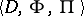, formed by the object domain, finite sets (signatures) of function,, and predicate,, symbols, with for each symbol its number of arguments (its arity) given.

The definition of the class of programs consists of three parts: the scheme of a program (its syntax), the interpretation and the semantics. The scheme of a program is a constructive object which indicates how the program is constructed using the signature and other formal symbols. The interpretation is the specification of a concrete object domain and the assignment to the signature symbols of concrete functions and predicates (basic operations), compatible with the object domain and the arities of the symbols. The semantics is a way of assigning to each program the result of executing it. As a rule, functions computable by a program are related to the program. The interpretation occurs as a parameter in the semantics, and therefore the scheme of a program defines a set of programs and functions computable by them, which can be obtained when varying the interpretation over some family of basic operations.

The scheme of a program with memory, also called an Algol-like or operator scheme, can be given as a finite oriented transition graph, with usually one input and one output vertex, and with vertices with one (transformers) and two (recognizers) output arcs. Using the signature symbols and a countable set of symbols for variables and constants one constructs in the usual way the set of function and predicate terms. Each recognizer is matched with some predicate term, while each transformer is matched with an assignment operator, of the formwhereis a variable anda function term. The finite set of variables in the scheme forms its memory. The interpretation in the complement to the concretization of the basic operations ascribes to each variable its extension domain and to each constant its value. So-called operational semantics is most common for programs with memory. It consists of an execution algorithm for the program on a given state of the memory. The program can be executed by movement over the transition graph. When hitting a recognizer one computes the predicate term and goes along an arc corresponding to the predicate value. When hitting a transformer with operator, one computes the value ofand ascribes it to. The result of execution of the program is the state of the memory when hitting the output vertex.

The scheme of a recursive program, or the recursive scheme, uses apart from function terms so-called conditional terms, which form together with the first the set of computable terms. A conditional term gives a computation by the method of sorting out cases and has the form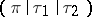, whereis a predicate and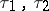are computable terms; it corresponds in Algol to the conditional statement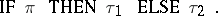The terms are constructed over the countable set of initial and formal variables, constants and symbols defining functions. A recursive scheme consists of a principal computable term with input variables and a finite family of recursive equations of the kind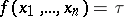, whereis the symbol for the function to be determined,are formal variables andis a term with variables from the set, while the functions to be determined belong to a family of equations. Under natural assumptions on the interpretation of the basic operations, the system of equations in the functions to be determined always has a so-called least fixed point (a set of functions, satisfying the equations, with graphs belonging to the graph of any other solution of these equations). Substituting the corresponding components of a least fixed point for the functions to be determined in the principal term, one obtains a function term, giving some function of the input variables which is also a function computable by the recursive program. Since such a manner of assigning to a program a function computable by it does not give a concrete computational algorithm, the semantics thus defined is called denotational.

The formalism indicated outlines, so to speak, the range of the level of expressive tools of programming languages: If the operator schemes are close to the structure of machine programs, then recursive schemes are close to the initial statement of the problem to be programmed.

Studies in theoretical programming bear the marks of the general mathematical tools used in investigating models of programs. The formal combinatorial methods form the theory of program schemes, which studies properties of a program that are invariant under the choice of an interpretation for the basic operations. By logical methods one studies means of defining the semantics of programs, and also searches for regularities in the process of constructing programs. In algebraic methods, which abstract from the concrete structure of a program, one directs one's attention to the investigation of sets arising when considering programs or classes of programs.

Outstanding branches of theoretical programming basically include models of sequential computations, performed by one active apparatus. The study of problems arising when it is necessary to organize the joint actions of a set of machines, unified in order to solve one problem or cooperating by means of signal transmission and information exchange, is the subject of a new discipline — so-called parallel programming.

## The theory of program schemes.

The fundamental concept of this theory is that of functional equivalence. Two schemes are called functionally equivalent if for any interpretation the corresponding programs compute identical functions. Every execution of a program can be assigned its protocol — a special kind of term in the signature of basic operations, reflecting the order of executing them. If the truth values of the predicates participating in the program are known, then the protocol can be uniquely constructed from these values, and in this construction one need not now the interpretation of the basic operations. If the truth values of predicates are selected arbitrarily, then as a result a certain set of formal protocols is created for the program scheme, called its determinant. Schemes are formally equivalent if their determinants coincide. Formal equivalence is correct if it implies functional equivalence. Since the determinant is constructed in a purely combinatorial way on the basis of an arbitrary selection out of a finite set, it forms a formal language accepted by some automaton. Definitions of protocols of program schemes that lead to solvable determinants are of special interest. For such determinants one can pose the problem of finding systems of transformations of program schemes that are complete in the sense that any two formally equivalent schemes can be translated into another by these transformations.

The structural theory of program schemes can be developed on the basis of the ground-laying work of A.A. Lyapunov and Yu.I. Yanov (cf. , ). The latter has completely investigated the simplest model of operator schemes with a signature of one-place operations and in which only one variable is admitted in the program (Yanov schemes). The protocol of a Yanov scheme is a sequence of executable operators, alternated by the values of predicates. As automaton accepting the determinant one may take a finite automaton; formal equivalence is solvable and, moreover, it coincides with functional equivalence. A complete system of transformations has been found for a Yanov scheme.

For general models of operator schemes, functional equivalence turns out to be unsolvable, but one has succeeded in finding a form of the protocol — the so-called logical-terminal history — which does lead to a solvable determinant. This protocol fixates the sequence of executions and values of the predicates of the scheme, while for each argument of the predicate it defines a functional term that would compute the value of the given argument when executing the program. As automaton accepting the determinant one may take a two-tape finite automaton. A complete system of transformations has been found for logical-terminal formal equivalence too.

The problems of translating program schemes from one computational model into another occupy an important place in the theory of program schemes. Operator schemes can be effectively translated into recursive schemes with the same signature, but the reverse translation is not possible, since the execution of a recursive program requires, in general, an arbitrary large number of memory cells.

The determinant of a recursive scheme can be specified as a context-free language (cf. Grammar, context-free), but the problem of solvability of the corresponding formal equivalence is as yet (1983) open.

The theory of program schemes also occupies itself with the study of individual classes of program schemes with the aim of singling-out the cases of a solvable equivalence; it also enriches the basic computational model by additional constructions of programming languages, studying moreover the expressional capabilities of the enrichment and reducibility problems.

## The logical theory of programs.

One says that a programis partially correct relative to an input conditionand output condition(denoted by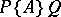) if, whenis true for the input values of the variables andhas terminated its operation,is true for the output values of the variables. Hereis called a pre-condition, anda post-condition, of. The programis called totally correct (denoted by) ifis partially correct relative toandand terminates its operation for input values of variables that satisfy. In order to prove that a sequential program is partially correct one often uses Floyd's method, which consists of the following. In the program scheme one choses control points such that any cyclic path passes through at least one point. The input and output of the scheme are also taken as control points. With every control point one associates a special condition (a so-called inductive statement or cycle invariant), which is true for every transition through this point. With the input and output points one associates input and output conditions. Now to every path of the program between two adjacent control points is assigned a so-called correctness condition. If all these conditions hold, the program must be partially correct. One way to prove that a program has terminated its operation consists in introducing additional counters into the program and in establishing the boundedness of these counters on the output of the program in the process of proving partial correctness.

It has been proposed to specify the semantics of programming languages axiomatically by means of a finite axiomatic system (a so-called Hoare logic), consisting of axioms and derivation rules in which statements on a program being partially correct are derivable as theorems. E.g., for the assignment operator the axiom scheme has the form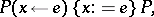where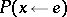denotes the result of substituting inthe expressionfor all occurrences of the variable, and for the cycle-type operator WHILE the derivation rule has the form(i.e.is a cycle invariant).

Suppose one considers the Hoare logic in which the language of first-order arithmetic is taken as the language for writing down the conditions. The statement that a program is partially correct is called derivable if it can be derived in an extension of this logic by means of adding true formulas of arithmetic, and it is called true if it is true with respect to the operational (or denotational) semantics of the program. A Hoare logic is called consistent if every statement that is derivable in it is also true, and it is called complete (with respect to arithmetic) if every true statement is derivable in it. A consistent and complete Hoare logic has been constructed, in particular, for programming languages containing simple variables, the assignment operator, compound and conditional operators, cycles and procedures (possibly recursive, but with a number of restrictions).

An important generalization of Hoare's logic is a so-called algorithmic (or dynamic) logic. Suppose thatcan be represented as, where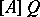is the weakest pre-condition: the statement that the programis partial correct with post-conditionis correct. Suppose that, in the case of total correctness, analogously,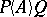can be represented as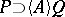. The formulas of algorithmic logic are constructed from the formulas of the basic logical language (for writing down conditions) and programs by means of the Boolean operations, quantifiers and also operations of the form,. Various statements about programs, e.g. their equivalence, can be expressed in algorithmic logic. Analogous to Hoare logic one has constructed for algorithmic logic a consistent and complete finite axiomatic system in the case of programming languages which also allow for non-deterministic programs.

In order to prove statements on recursive programs one often uses a special induction, related with the definition of a least fixed point. Suppose, for simplicity, that a recursive program is given by one equation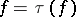and that its denotational semantics is a least fixed point. Under natural assumptions regarding the conditionthe following induction principle holds: If a formulais true and ifimplies, then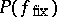is fulfilled (here,is a nowhere-defined function). In order to describe the denotational semantics of higher-level programming languages one uses the specification of the domain of data as a so-called complete Scott lattice.

The problem of synthesis of a program can be formalized as the problem of constructing a proof of a theorem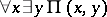with subsequent extraction of a program from this proof. One has constructed an algorithm that, from a proof in intuitionistic logic, gives a program in Algol-68. If the proof uses an induction rule, then a cycle of the form FORTODOOD corresponds to it in the program. The problem extracted is of admissible complexity if one uses the assumption that it is constructed from standard (i.e. given) functions and predicates, whose properties are described by axioms of a special form.

## The algebraic theory of programs.

As an example of the use of algebraic methods in theoretical programming one may take Glushkov's problem of equivalence of discrete transformers, in which operator program schemes can be naturally included. Letbe a finite Mealey automaton with input alphabetand output alphabetand with given initial and final states (cf. Automaton, finite). Letbe the semi-group with set of generatorsand identity. Consider the (possibly infinite) Moore automaton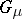with set of states, inputs, outputs, initial state, output function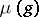, and transition function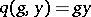. The automaton, functioning compatible with, is called a discrete transformer ifaccepts the output ofas input and ifaccepts the output ofas input. An output ofis, here, the state ofat the halting moment of. Discrete transformers are equivalent with respect to the semi-groupif for every mappingthey both do not halt together withor if they both halt with identical output. One has established the solvability of the problem of equivalence of discrete transformers with respect to a left-cancellation semi-group in which the identity is indecomposable and in which the word (identity) problem is solvable. One has also described all solvable and unsolvable cases of equivalence of discrete transformers with respect to a commutative semi-group.

Theoretical programming shows its influence in practice most of all as a conceptual frame for the study of programming and — in a more technical way — through the theory of formal programming languages. Here, properties of abstract computational models are used for refining the semantics of programming languages and for providing a foundation for various manipulations with programs (cf. Translation of programs; Program-optimizing transformations).